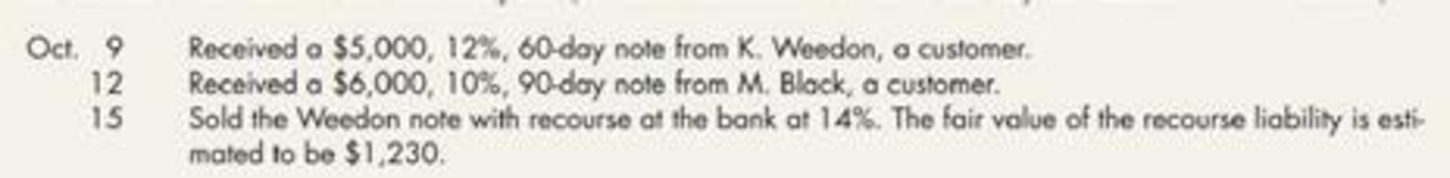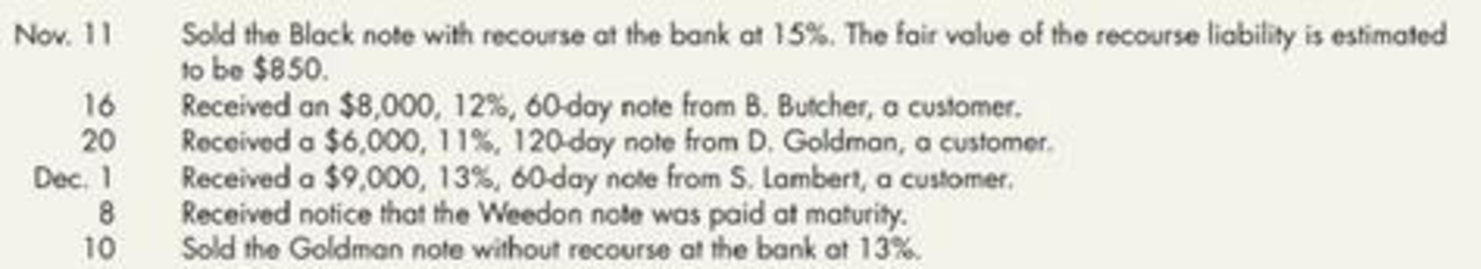Chapter 6, Problem 13P### Intermediate Accounting: Reporting...

3rd Edition
James M. Wahlen + 2 others
ISBN: 9781337788281

#### Solutions

Chapter
Section### Intermediate Accounting: Reporting...

3rd Edition
James M. Wahlen + 2 others
ISBN: 9781337788281
Textbook Problem
83 views

# Notes Receivable Transactions The following notes receivable transactions occurred for Harris Company during the last three months of the current year. (Assume all notes are dated the day the transaction occurred.)Required: 1. Prepare the journal entries to record the preceding note transactions and the necessary adjusting entries on December 31. (Assume that Harris does not normally sell its notes and uses a 360-day year for the purpose of computing interest. Round all calculations to the nearest penny.) 2. Show how Harris’ notes receivable would be disclosed on the December 31 balance sheet. (Assume these are the only note transactions encountered by Harris during the year.)

1.

To determine

Record journal entries for previous note transactions and prepare the adjusting entries.

Explanation

Note receivable:

Note receivable refers to a written promise for the amounts to be received within a stipulated period of time. This written promise is issued by a debtor or, borrower to the lender or creditor. Notes receivable is an asset of a business.

Accounts receivable:

Accounts receivable refers to the amounts to be received within a short period from customers upon the sale of goods and services on account. In other words, accounts receivable are amounts customers owe to the business. Accounts receivable is an asset of a business.

 Date Account Title and Explanation Debit Credit December 1 Notes receivable $9,000 Accounts receivable$9,000 (To record the notes receivable)

Table (1)

• Notes receivable is an asset and it is increased. Therefore, debit notes receivable account by $9,000. • Accounts receivable is an asset and it is decreased. Therefore, credit accounts receivable account by$9,000.
 Date Account Title and Explanation Debit Credit December 8 Recourse liability $1,230 Gain from sale of notes$1,230 (To record the fair value of recourse liability)

Table (2)

• Recourse liability is a liability and it is decreased. Therefore, debit recourse liability account by $1,230. • Gain from sale of notes is a component of stockholders’ equity and it is increased. Therefore, credit gain from sale of notes account by$1,230.
 Date Account Title and Explanation Debit Credit December 10 Cash (1) $5,995.39 Loss from sale of receivable (1)$41.28 Notes receivable $6,000 Interest income (5)$36.67 (To record note discounted on November 20 )

Table (3)

• Cash is an asset and it is increased. Therefore, debit cash account by $5,995.39 • Loss from sale of receivable is a component of stockholders’ equity and it is decreased. Therefore, debit loss from sale of receivables by$41.28
• Notes receivable is an asset and it is increased. Therefore, credit notes receivable account by $6,000. • Interest income is a component of stockholders’ equity and it is increased. Therefore, credit interest income account by$36.67.
 Date Account Title and Explanation Debit Credit December 31 Interest receivable $217.50 Interest income(6)$217.50 (To record the interest income of note)

Table (4)

• Interest receivable is an asset and it is increased. Therefore, debit interest receivable account by $217.50. • Interest income is a component of stockholders’ equity and it is increased. Therefore, credit interest income account by$217.50.

Working note:

(1) Calculate the amount of loss from receivable:

 Particulars Amount ($) Face value of the note 6,000 Interest to maturity (2) 220 Maturity value of note 6,220 Less: Discount (3) ($224.61) Proceeds 5,995.39 Less: Book value of note (4) (6,036.67) Loss from sale of receivable 41.28

Table (5)

(2) Calculate the interest to maturity:

Interesttomaturity=(NotereceivableonNovember20×Percentageofinterest×Timeperiod)=$6,000×11%×120days360days=$660×120days360days=$220 (3) Calculate the amount of discount: Discount=(Maturityvalueofnote×percentageofinterest×Timeperiod)=$6,220×13%×(12020360days)=\$808

2.

To determine

State the manner in which the notes receivable of Company H will be disclosed on the balance sheet on December 31.

### Still sussing out bartleby?

Check out a sample textbook solution.

See a sample solution

#### The Solution to Your Study Problems

Bartleby provides explanations to thousands of textbook problems written by our experts, many with advanced degrees!

Get Started

#### Find more solutions based on key concepts